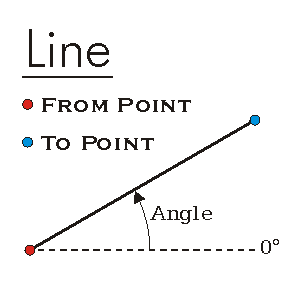This document is archived and information here might be outdated.  Recommended version.

ILine Interface (ArcObjects .NET 10.8 SDK)
 ArcObjects Help for .NET developers > ArcObjects Help for .NET developers > ArcObjects namespaces > Geometry > ESRI.ArcGIS.Geometry > Interfaces > IL > ILine Interface
 ArcGIS Developer Help

# ILine Interface

Provides access to members that identify a straight line segment and defines its properties. Note: the ILine interface has been superseded by ILine2. Please consider using the more recent version.

#### Product Availability

Available with ArcGIS Engine, ArcGIS Desktop, and ArcGIS Server.

#### Members

Name DescriptionAngle The angle between this line and the positive x-axis.Dimension The topological dimension of this geometry.Envelope Creates a copy of this geometry's envelope and returns it.FromPoint The 'from' point of the curve.GeometryType The type of this geometry.GeoNormalize Shifts longitudes, if need be, into a continuous range of 360 degrees.GeoNormalizeFromLongitude Normalizes longitudes into a continuous range containing the longitude. This method is obsolete.GetSubcurve Extracts a portion of this curve into a new curve.IsClosed Indicates if 'from' and 'to' points (of each part) are identical.IsEmpty Indicates whether this geometry contains any points.Length The length of the curve.Project Projects this geometry into a new spatial reference.PutCoords Sets this line's endpoints to be 'from' and 'to'.QueryCoords Copies the endpoints of this line to 'from' and 'to'.QueryEnvelope Copies this geometry's envelope properties into the specified envelope.QueryFromPoint Copies this curve's 'from' point to the input point.QueryNormal Constructs a line normal to a curve from a point at a specified distance along the curve.QueryPoint Copies to outPoint the properties of a point on the curve at a specified distance from the beginning of the curve.QueryPointAndDistance Finds the point on the curve closest to inPoint, then copies that point to outPoint; optionally calculates related items.QueryTangent Constructs a line tangent to a curve from a point at a specified distance along the curve.QueryToPoint Copies the curve's 'to' point into the input point.ReverseOrientation Reverses the parameterization of the curve ('from' point becomes 'to' point, first segment becomes last segment, etc).SetEmpty Removes all points from this geometry.SnapToSpatialReference Moves points of this geometry so that they can be represented in the precision of the geometry's associated spatial reference system.SpatialReference The spatial reference associated with this geometry.ToPoint The 'to' point of the curve.

#### Inherited Interfaces

Interfaces Description
ICurve Provides access to properties and methods of all 1 dimensional curves (polylines, segments, boundaries of polygons, etc.).
IGeometry Provides access to members that describe properties and behavior of all geometric objects.

#### Classes that implement ILine

Classes Description
Line A 2D straight line between a pair of 2D endpoints; can optionally have height, measure and ID attributes at each endpoint.

#### Remarks

The PutCoords method requires 2 Point objects to construct the line segment.[C#]

// This example creates a simple line and gets the angle
// and the start and endpoint.
public void ShowLineAngle()
{
IPoint fromPoint = new PointClass();
fromPoint.PutCoords(100, 100);

IPoint toPoint = new PointClass();
toPoint.PutCoords(150, 150);

ILine line = new LineClass();
line.PutCoords(fromPoint, toPoint);
double angle = line.Angle;

//query Coordinats
IPoint outFromPoint = new PointClass();
IPoint outToPoint = new PointClass();
line.QueryCoords(outFromPoint, outToPoint);

System.Windows.Forms.MessageBox.Show("From X = " + outFromPoint.X +
", From Y = " + outFromPoint.Y + "\n" +
"To X = " + outToPoint.X +
", To Y = " + outToPoint.Y + "\n" +
"Angle = " + angle);
}